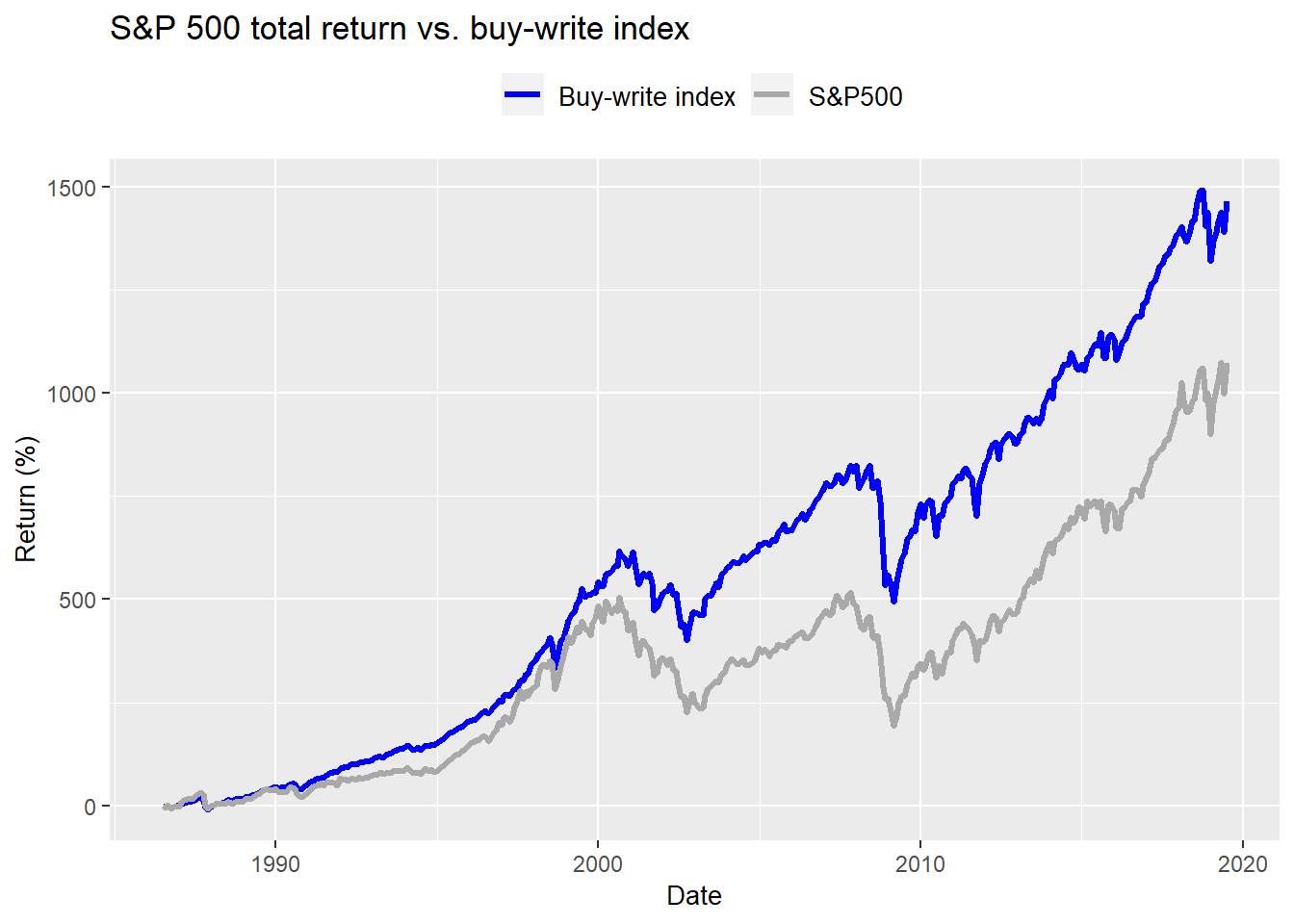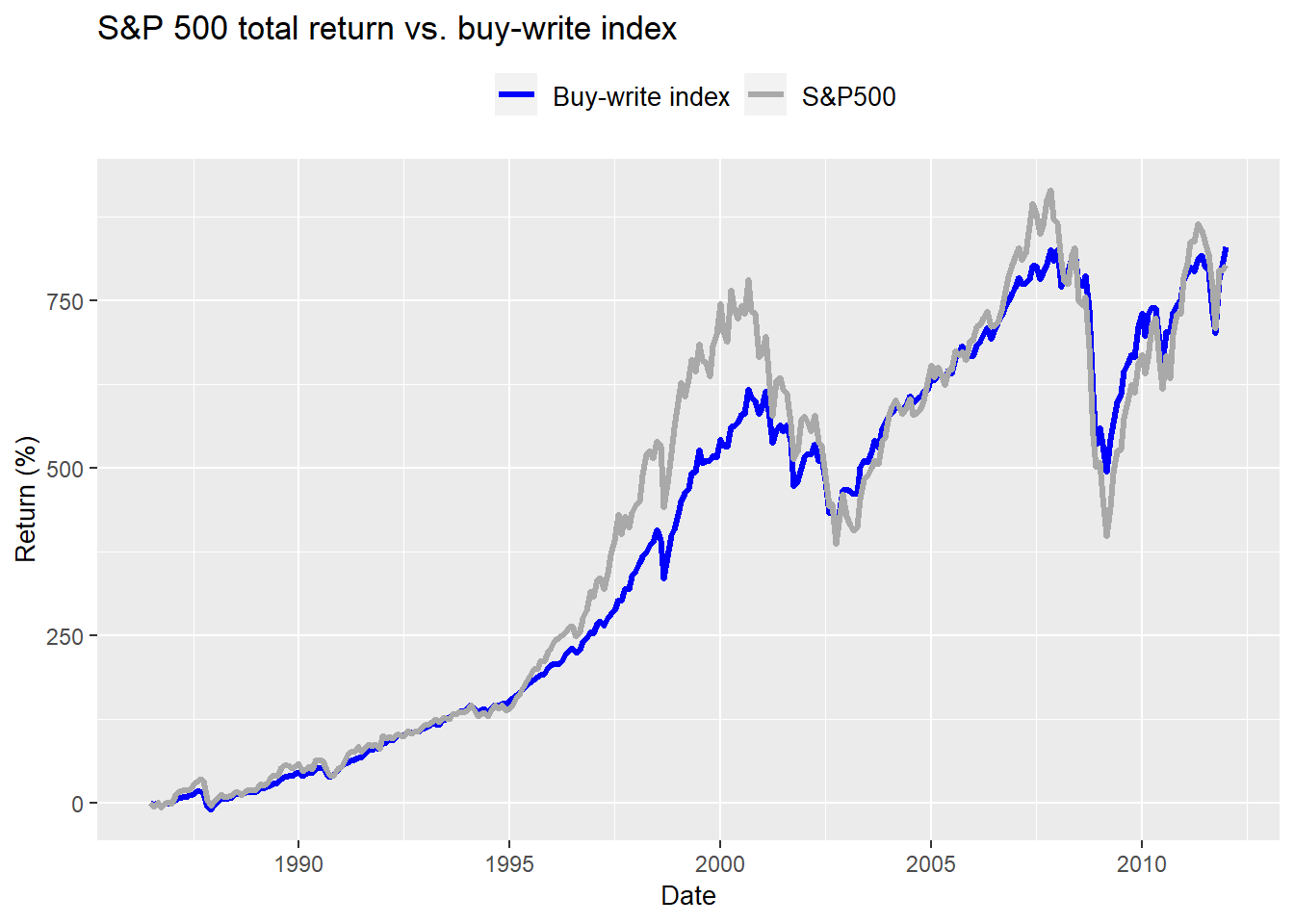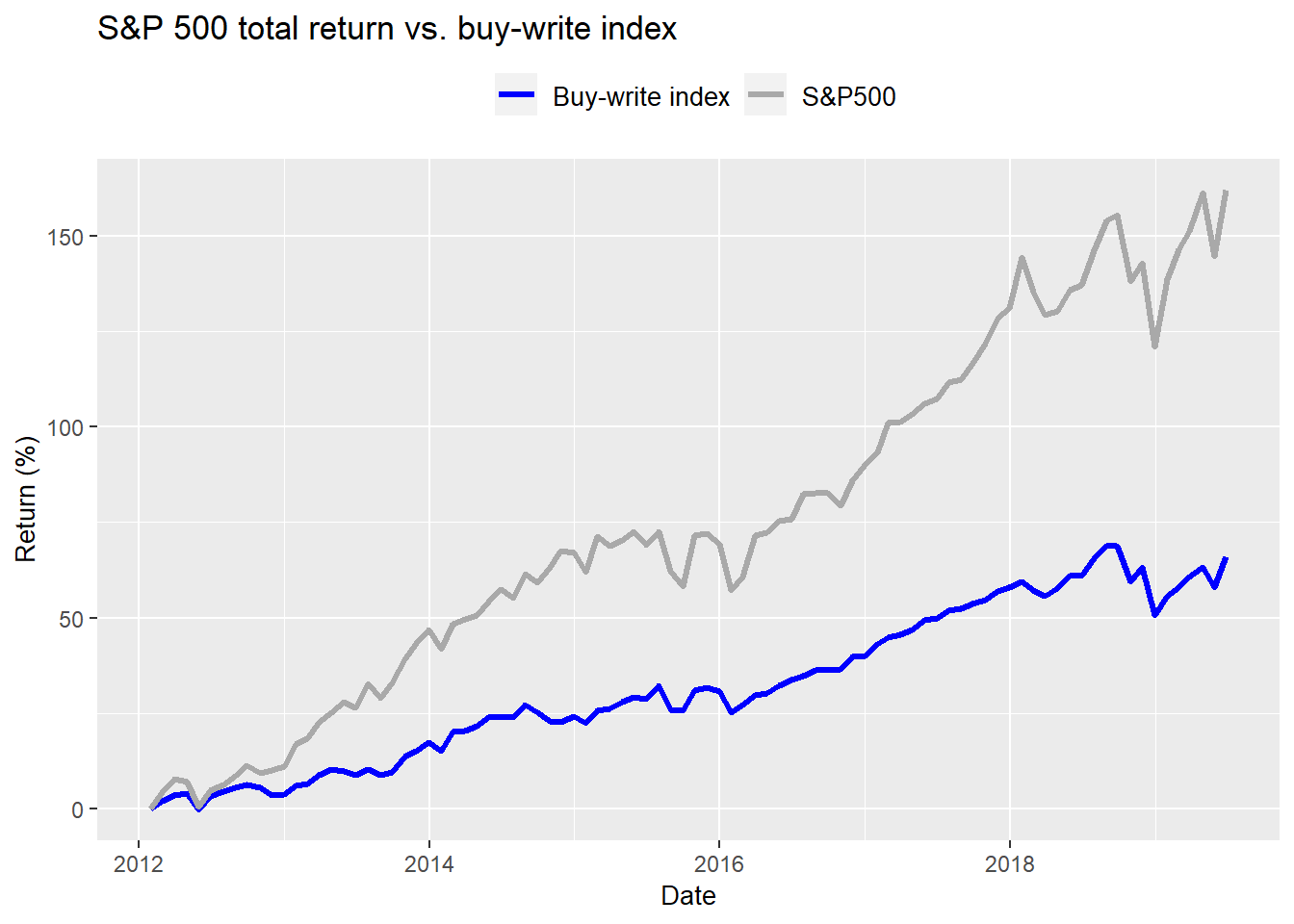# Calling covered data

In our last post on covered calls we introduced the CBOE’s buy-write index (or BXM), whose underlying is the S&P500 index. We looked at some of the historical data, made a few comparisons between the index and the S&P, and noted that there was a report that analyzed the buy-write index. In this post, we’ll look at some of the findings from that report, which can be found on the CBOE’s website.

The key findings we’ll examine are:

• Total growth: 830% (9.1% annualized) for BXM Index vs. 807% (9.0% annualized) for S&P 500
• Lower Volatility: BXM had volatility that was about 30% lower than the S&P 500
• Risk-adjusted Returns: Using the Sharpe Ratio (we’ll explain later), risk-adjusted returns were 0.52 for BXM and 0.30 for S&P 500
• Left-tail risk: the biggest monthly loss for the S&P was 16.8%, while it was 15.1% for the BXM

Let’s first verify these results, and then compare them to the period after the report was published as well as the total history to see if there’s anything we can see. The data covered the period from June 30, 1986 to December 31, 2011.

As a refresher here’s the graph of the total return of the BXM and the S&P 500.Now let’s look at it for the period of interest—through the end of 2011.Can we verify the results? For the most part we can. There’s a small difference in the total return on the S&P, and volatilities on both indices, but the differences are less than 1%, so not material.

Table 1: Strategy summary results
Strategy Total return (%) Annualized return (%) Volatility (%)
BXM 829.9 9.1 11.6
S&P 500 803.2 9.0 16.1

What about left-tail risk? According to the report, the worst monthly returns for the BXM and the S&P were 15.1% and 16.8%. We were not able to replicate those figures. Our calculations suggest results much worse than reported in the findings.

Table 2: Left tail risk
Strategy Maximumn monthly loss (%)
BXM -19.2
S&P 500 -24.3

The cause appears to be that the report analyzed left tail risk only on data from June 1988. That excludes the 1987 period, which featured Black Monday when the S&P 500 fell over 20% in one day. If you include the full period on which the rest of the report is based, you find that October 2008, which the report highlights as the worst month, was, in fact, the second worst. we show the top five worst monthly returns in the table below for the full 1986 to 2011 data series.

Table 3: Top five worst monthly returns (%)
Date BXM S&P 500
1987-10-30 -19.2 -24.3
2008-10-31 -16.4 -18.4
1998-08-31 -12.6 -15.6
2008-11-28 -11.3 -7.4
2001-09-28 -11.1 -8.4

Even so, the figures calculated for the October 2008 returns are about 130-160bps worse than the report. It is unclear what’s causing the difference, but it isn’t worth the time to figure that out. Indices are often revised or adjusted after the fact, which might be the issue here.

The more interesting issue is to compare what the data suggest about the attractiveness of the strategy at the time the report came out compared to what occurred aftereward. While the report does not recommend investing in the various strategies analyzed, it’s hard to discount the implications. That is, if the BXM outperforms the S&P 500 and does so with lower risk, why wouldn’t it make sense to include the BXM strategy in a portfolio? But does this performance persist? The following chart shows the cumulative returns since 2011.Not exactly a great result. What do the actual numbers look like? The S&P’s performance was over two times better on a cumulative basis. True, volatility was lower.

Strategy Total return (%) Annualized return (%) Volatility (%)
BXM 66.0 2.0 7.2
S&P 500 161.9 3.8 11.5

Of course, it is not entirely fair to simply look at the returns and volatility. One needs to look at risk-adjusted returns. Most investors use the Sharpe or Sortino ratios. We use return-to-risk, which is simply the Sharpe ratio where the risk-free rate equals zero.1

How do the risk-adjusted returns compare? Not very well. As we see in the table below in the pre-2012 period, risk-adjusted returns for the BXM significantly outperform the S&P 500. After 2012, performance completely flips by almost the same magnitude from over 20 points of outperformance to 20 points of underperformance. That is a dramatic reversal!

Table 4: Return-to-risk (%) in and out of sample
Sample BXM S&P 500
1986-2012 75.2 53.6
2012-Present 94.4 113.4

What caused such a reversal? The mathematical cause is that returns to the BXM were capped due to the options. And the nuance is that the option seller wasn’t being adequately compensated for the reduced upside potential. At least that’s what appears to be the case on first blush. But those conjectures don’t answer the fundamental why? That is, why did the S&P perform so well and/or why weren’t option sellers compensated adequately? Answering those questions would take longer than a couple weeks worth of blog posts. Probably even a couple months.

But here are a couple of ideas. On the S&P’s performance, historically low interest rates, accomodative fiscal policy, robust GDP growth, and greater risk-taking are some places to start. On options premia being too low, possible explanations are skepticism around market upside and market knowledge of studies that tout selling calls! We surmise the second conjecture may have had the most impact, even though we don’t have the data to prove it.2 The logic is, if more investors are implementing a covered call strategy, then premia should decline as incremental demand to sell should equate to lower offers to buy (the other side of the trade), all else equal.

Whatever the case, what is the takeaway? Past performance is not a predictor of future results? The lame warning you see on every mutual fund prospectus? A better question is, would one have expected to see performance diverge this much based on the data up to and including 2011? To answer that, we’ll need to return to what we learned about simulating stock returns in our post on diversification, which we’ll save for another time.

As a teaser, on a simple simulation we find that 46.1% of the time the BXM underperformed the S&P on a cumulative basis given the historical data. But it’s risk-adjusted returns were worse only about 32.1% of the time. Still, the chance that the buy-write strategy would produce such poor risk-adjusted results seems surprisingly high given that’s it’s supposed to offset some of the downside. We’ll need to investigate that further and look into whether the severity of the underperformance on an absolute and risk-adjusted basis would have been expected.

Until then, here is all the code used to generate the graphs and analysis.

# Load packages
library(tidyquant)

# Graph return of buy-write vs S&P
cboe %>%
gather(key, value, -date) %>%
# mutate(value = as.numeric(value)) %>%
filter(key %in% c("bxm", "spx")) %>%
group_by(key) %>%
mutate(value = (value/value-1)*100) %>%
ggplot(aes(date, value, color = key)) +
geom_line(lwd = 1.1) +
scale_color_manual("", labels = c("Buy-write index", "S&P500"),
values = c("blue", "darkgrey"))+
theme(legend.position = "top",
legend.text = element_text(size = 10),
axis.title = element_text(size = 10)) +
labs(x = "Date",
y = "Return (%)",
title = "S&P 500 total return vs. buy-write index")

# Returns to 2011
cboe %>%
gather(key, value, -date) %>%
# mutate(value = as.numeric(value)) %>%
filter(key %in% c("bxm", "sptr")) %>%
group_by(key) %>%
filter(date <= "2012-01-01") %>%
mutate(value = (value/value-1)*100) %>%
ggplot(aes(date, value, color = key)) +
geom_line(lwd = 1.1) +
scale_color_manual("", labels = c("Buy-write index", "S&P500"),
values = c("blue", "darkgrey"))+
theme(legend.position = "top",
legend.text = element_text(size = 10),
axis.title = element_text(size = 10)) +
labs(x = "Date",
y = "Return (%)",
title = "S&P 500 total return vs. buy-write index")

# Table
cboe %>%
gather(Strategy, value, -date) %>%
filter(Strategy %in% c("bxm", "sptr"),
date <= "2012-01-01") %>%
mutate(Strategy = case_when(Strategy == "bxm" ~ "BXM",
Strategy == "sptr" ~ "S&P 500")) %>%
group_by(Strategy) %>%
mutate(return = ROC(value)) %>%
summarise(Total return (%) = round((last(value)/first(value)-1),3)*100,
Annualized return (%) = round((last(value)/first(value))^(1/25.58)-1,3)*100,
Volatility (%) = round(sd(return, na.rm = TRUE) * sqrt(12),3)*100) %>%
knitr::kable("html", caption = 'Strategy summary results')

# Table
cboe %>%
gather(Strategy, value, -date) %>%
filter(Strategy %in% c("bxm", "sptr"),
date <= "2012-01-01") %>%
mutate(Strategy = case_when(Strategy == "bxm" ~ "BXM",
Strategy == "sptr" ~ "S&P 500")) %>%
group_by(Strategy) %>%
mutate(return = ROC(value)) %>%
summarise(Maximumn monthly loss (%) = round(min(return, na.rm = TRUE),3)*100) %>%
knitr::kable(caption = "Left tail risk")

# Table
# Top 5 worst
cboe %>%
gather(key, value, -date) %>%
group_by(key) %>%
mutate(return = 100*round(ROC(value),3)) %>%
filter(key %in% c("bxm", "sptr")) %>%
select(-value) %>%
arrange(bxm) %>%
rename("Date" = date,
"BXM" = bxm,
"S&P 500" = sptr) %>%
knitr::kable(caption = "Top five worst monthly returns (%) ")

### After 2011
cboe %>%
gather(key, value, -date) %>%
# mutate(value = as.numeric(value)) %>%
filter(key %in% c("bxm", "sptr")) %>%
group_by(key) %>%
filter(date >= "2012-01-01") %>%
mutate(value = (value/value-1)*100) %>%
ggplot(aes(date, value, color = key)) +
geom_line(lwd = 1.1) +
scale_color_manual("", labels = c("Buy-write index", "S&P500"),
values = c("blue", "darkgrey"))+
theme(legend.position = "top",
legend.text = element_text(size = 10),
axis.title = element_text(size = 10)) +
labs(x = "Date",
y = "Return (%)",
title = "S&P 500 total return vs. buy-write index")

# Table
cboe %>%
gather(Strategy, value, -date) %>%
filter(Strategy %in% c("bxm", "sptr"),
date >= "2012-01-01") %>%
mutate(Strategy = case_when(Strategy == "bxm" ~ "BXM",
Strategy == "sptr" ~ "S&P 500")) %>%
group_by(Strategy) %>%
mutate(return = ROC(value)) %>%
summarise(Total return (%) = round((last(value)/first(value)-1),3)*100,
Annualized return (%) = round((last(value)/first(value))^(1/25.5)-1,3)*100,
Volatility (%) = round(sd(return, na.rm = TRUE) * sqrt(12),3)*100) %>%
knitr::kable()

# Risk adjusted before and after
cboe %>%
gather(Strategy, value, -date) %>%
filter(Strategy %in% c("bxm", "sptr")) %>%
mutate(Strategy = case_when(Strategy == "bxm" ~ "BXM",
Strategy == "sptr" ~ "S&P 500"),
Sample = ifelse(date <= "2012-01-01", 1, 2)) %>%
group_by(Strategy, Sample) %>%
mutate(return = ROC(value)) %>%
summarise(return = round(mean(return, na.rm = TRUE)/
sd(return, na.rm = TRUE) * sqrt(12),3)*100) %>%
mutate(Sample = recode(Sample,
"1"  = "1986-2012",
"2" = "2012-Present")) %>%
knitr::kable(caption = "Return-to-risk (%) in and out of sample")

# Get mean and std dev
num <- cboe %>%
filter(date < "2012-01-01") %>%
nrow()

bxm_mean <- mean(ROC(cboe$bxm[1:num]), na.rm = TRUE) bxm_sig <- sd(ROC(cboe$bxm[1:num]), na.rm = TRUE)

sptr_mean <- mean(ROC(cboe$sptr[1:num]), na.rm = TRUE) sptr_sig <- sd(ROC(cboe$sptr[1:num]), na.rm = TRUE)

# Create simulation function
cum_ret <- function(mu, sigma){
returns <- rnorm(90, mu, sigma)
cum_returns <- prod(returns+1)-1
ret_risk <- mean(returns)/sd(returns)
lt <- data.frame(ret_risk = ret_risk, cum_returns = cum_returns)
lt
}

# Simulate
set.seed(123)
bxm_sim <- plyr::rdply(1000, cum_ret(bxm_mean, bxm_sig))
sp_sim <- plyr::rdply(1000, cum_ret(sptr_mean, sptr_sig))

# Likelihood bxm performs worse than sp
prob_upf <- round(mean(bxm_sim$cum_returns < sp_sim$cum_returns),3)*100
prob_poor_risk <- round(mean(bxm_sim$ret_risk < sp_sim$ret_risk),3)*100

1. We use a zero risk-free rate because it’s more reproducible. No one who’s reading this will need to figure out which risk-free rate we used. Second, risk-free rates change over time, which means you need to have the right data series and the right time period adjustments to have a robust series. Third, we tried to layer in the risk-free rate, but was not able to reproduce the results. Trying to do that or explaining the issues around that hurdle are beyond the scope of this blog. Apologies to the purists.

2. One could look at call option volume prior to and after the report date and scale it relative overall option volume as an initial way to prove that assertion.